# explore-many-variables

|   Source

In this Lesson we want to add 3 or more variables to observe. We also want to use third another variable to look some consistency of 2 variables we want to observe.

### Moira Perceived Audience Size Colored by Age

• Moira then observe the the audience size, and come with another question
• is it older people better than younger people in estimate the audience?
• so she began to plot the age based on color. But it doesn’t help much ***

### Third Qualitative Variable

• In Moira’s experiment, she didn’t have any correlation of age and the audience size.
• In this experiment, we want to find the correlation between age and gender.
• Here we can see that women’s average get higher percentage of friend_count then it is for men
• And also notice froom boxplot, that women has more number, with median beyond 30
• next we want to group_by two variable by using dplyr, groupby, summarise, and arrange
``````library(ggplot2)
ggplot(aes(x = gender, y = age),
data = subset(pf, !is.na(gender))) +geom_boxplot()+
stat_summary(fun.y = mean, geom = "point", shape =4 )``````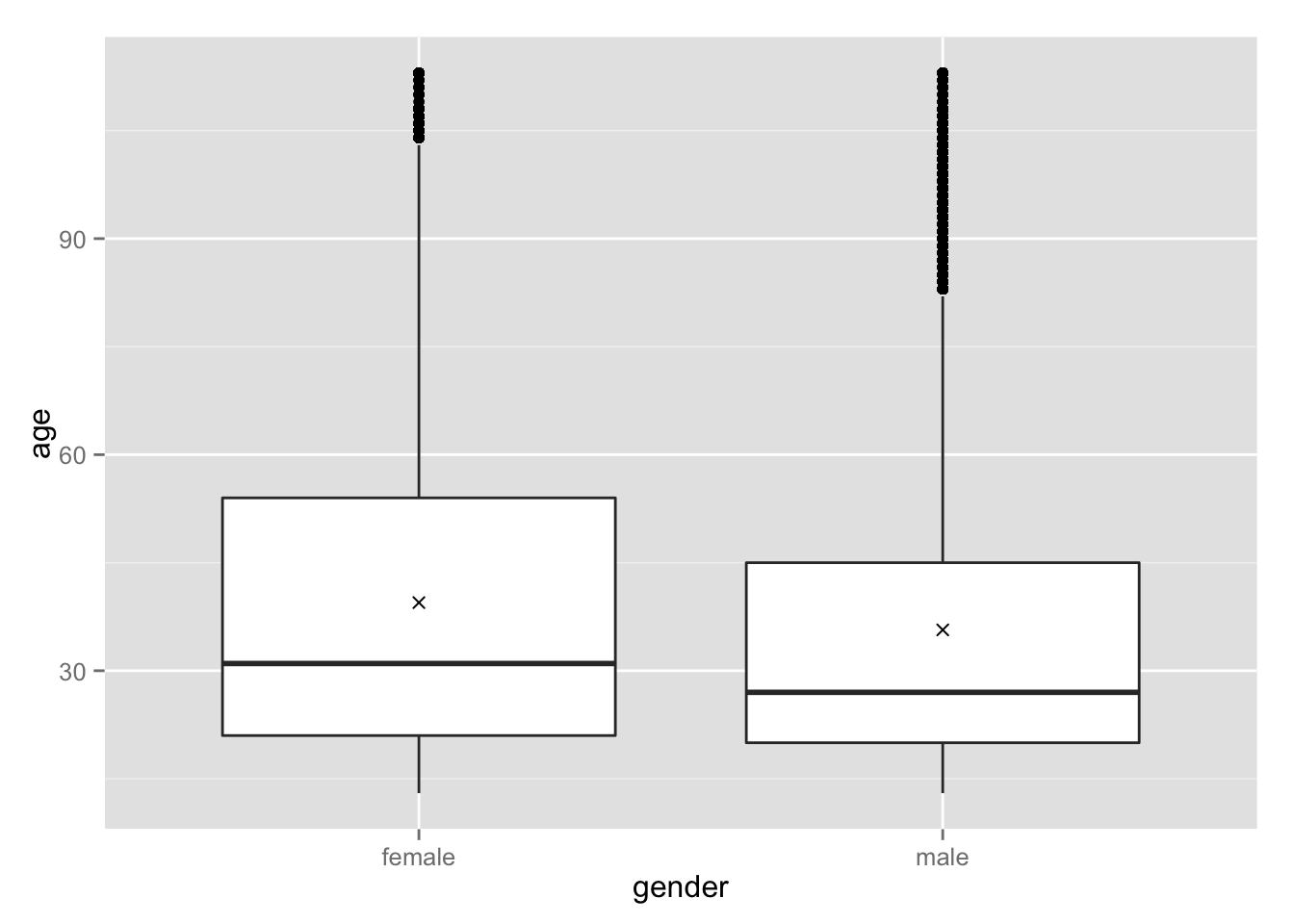``````#+ geom_histogram()

ggplot(aes(x = age, y = friend_count),
data= subset(pf, !is.na(gender)))+
geom_line(aes(color=gender), stat="summary", fun.y = median)``````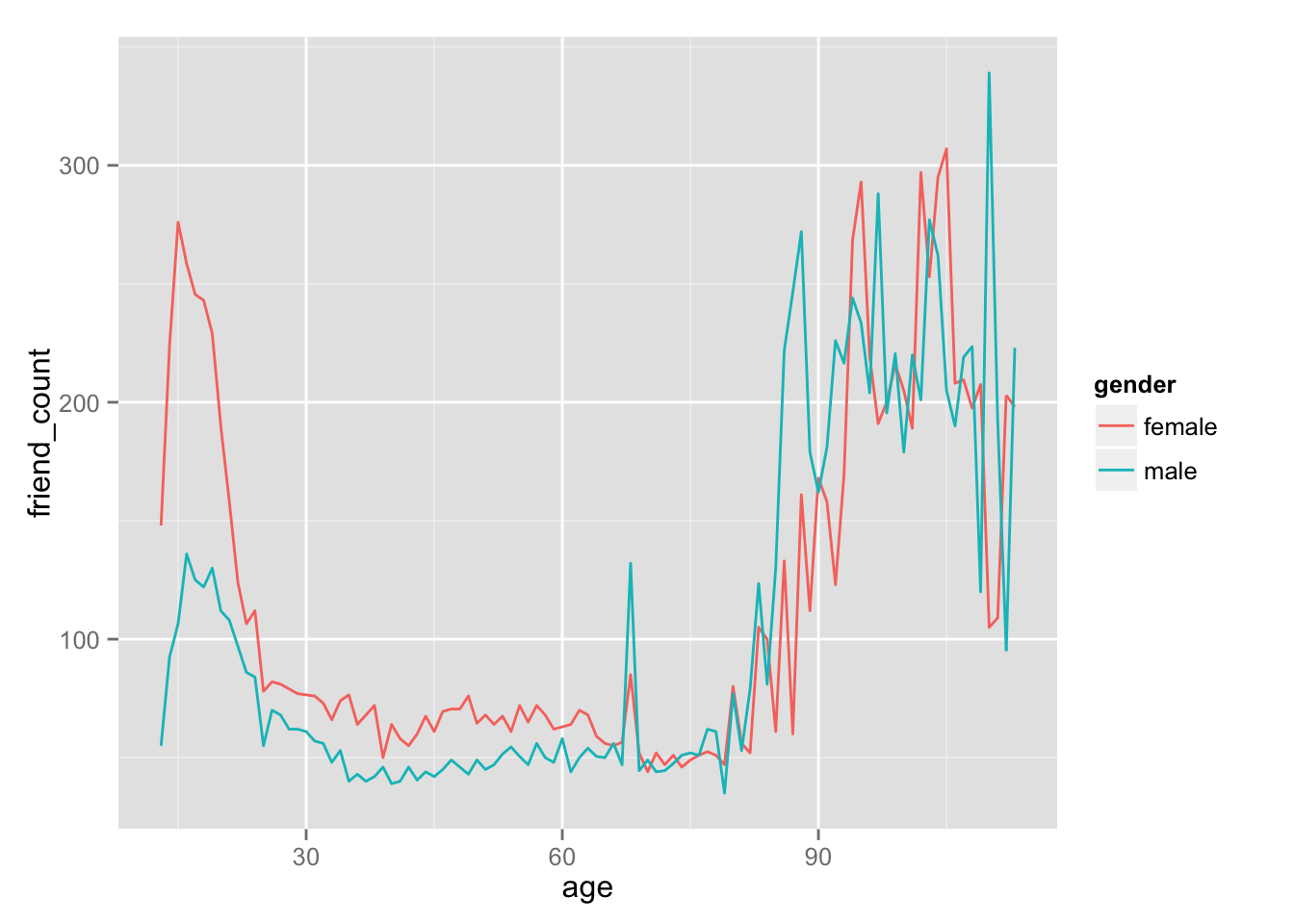``library(dplyr)``
``````##
## Attaching package: 'dplyr'
##
## The following object is masked from 'package:stats':
##
##     filter
##
## The following objects are masked from 'package:base':
##
##     intersect, setdiff, setequal, union``````
``````pf.fc_by_age_gender <- group_by(pf,age,gender) %>%
filter(!is.na(gender))%>%
summarise(median_friend_count = median(friend_count),
mean_friend_count = mean(friend_count),
n=n())%>%
#Earlier we use groupby age,gender. because gender need to be avoided, remove one layer
#by using ungroup, and arrange by age
ungroup()%>%
arrange(age)
``````## Source: local data frame [6 x 5]
##
##   age gender median_friend_count mean_friend_count    n
## 1  13 female                 148          259.1606  193
## 2  13   male                  55          102.1340  291
## 3  14 female                 224          362.4286  847
## 4  14   male                  92          164.1456 1078
## 5  15 female                 276          538.6813 1139
## 6  15   male                 106          200.6658 1478``````

### Plotting Conditional Summaries

Create a line graph showing the median friend count over the ages for each gender. Be sure to use the data frame you just created, pf.fc_by_age_gender. Instructor Notes

Your code should look similar to the code we used to make the plot the first time. It will not need to make use of the stat and fun.y parameters.

ggplot(aes(x = age, y = friend_count), data = subset(pf.1, !is.na(gender))) + geom_line(aes(color = gender), stat = ‘summary’, fun.y = median)

``````ggplot(aes(x= age, y=median_friend_count),
data = pf.fc_by_age_gender)+
geom_line(aes(color=gender))``````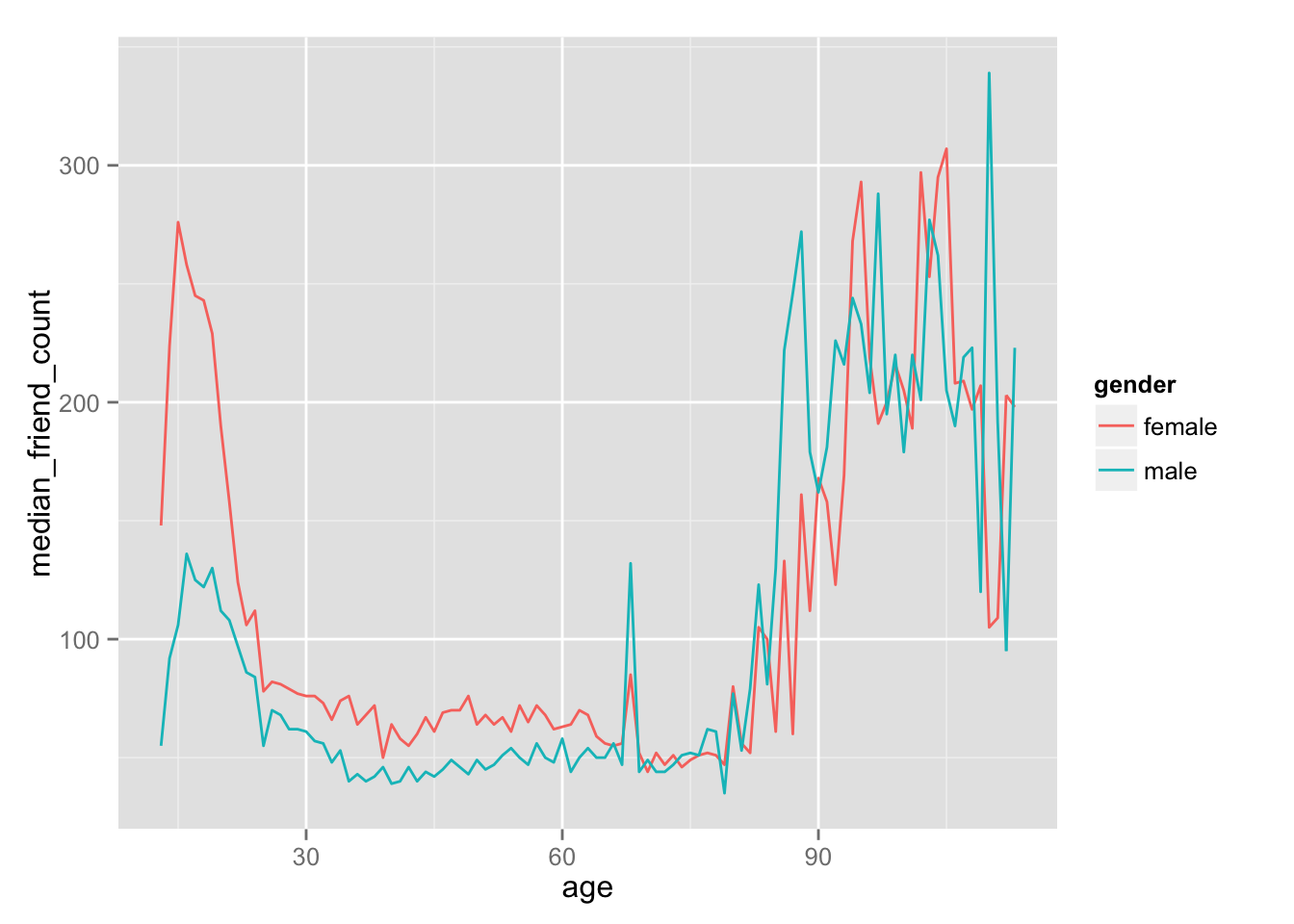### Thinking in Ratios

• Now by this plot we know plotting in range of ages with different gender.
• We also spot that younger people tend to have more friend.
• Now we may want to ask different question. By how many ratio women have friend compare to men?

### Wide and Long Format

• By doing this, we want to reshape our data into different format.
• Notice that our subset of data have repeated age.
• Now we want to reshape our data, into wide format.
• one row each age, put median value inside male and female
• It’s normal to be back and forth with the data in different arrangement.
• To do this, we’re using ‘reshape’ packages.
• Similar to octave, we’re reshaping from wide<->long depending on what we do.
• wide(multiple) column to long row, or the other way around

### Reshaping Data

It???s important to use quotes around the variable name that is assigned tovalue.var.

We could also create a similar data frame using the dplyr package. pf.fc_by_age_gender.wide <- pf.fc_by_age_gender %.% group_by(age) %.% summarise(male = friend_count.median[gender = ‘male’], female = friend_count.median[gender = ‘female’], ratio = female / male) %.% arrange(age)

``````library(reshape2)
pf.fc_by_age_gender.wide <- dcast(pf.fc_by_age_gender,
age~gender,#formula,left=value that kept,right=column that retain
value.var='median_friend_count')
``````##   age female male
## 1  13    148   55
## 2  14    224   92
## 3  15    276  106
## 4  16    258  136
## 5  17    245  125
## 6  18    243  122``````

### Ratio Plot

Plot the ratio of the female to male median friend counts using the data frame pf.fc_by_age_gender.wide.

Think about what geom you should use. Add a horizontal line to the plot with a y intercept of 1, which will be the base line. Look up the documentation for geom_hline to do that. Use the parameter linetype in geom_hline to make the line dashed.

The linetype parameter can take the values 0-6: 0 = blank, 1 = solid, 2 = dashed 3 = dotted, 4 = dotdash, 5 = longdash 6 = twodash

``````library(ggplot2)
ggplot(aes(x=age, y = female/male),
data=pf.fc_by_age_gender.wide)+
geom_line()+
geom_hline(aes(yintercept=1),linetype=2)``````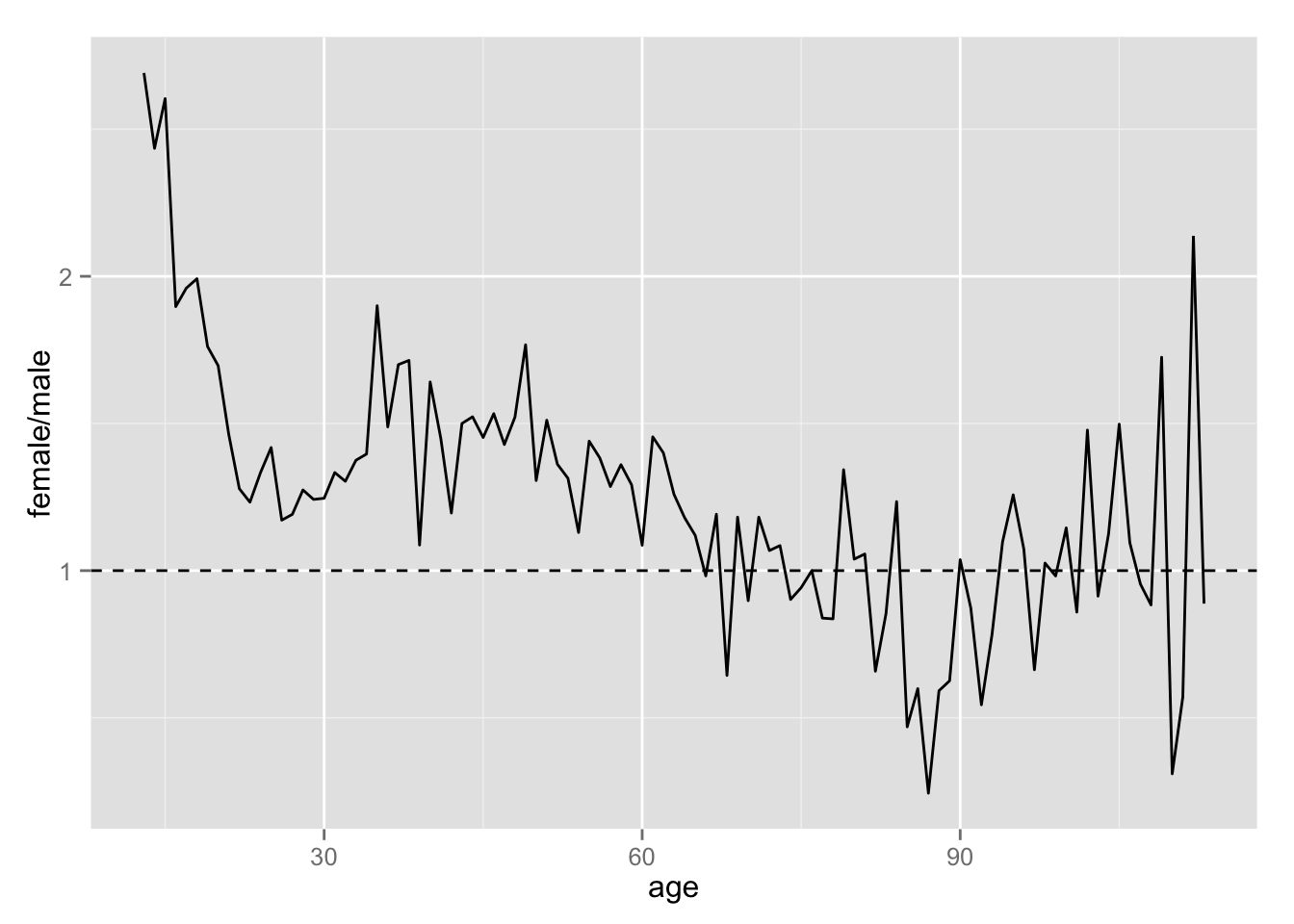Pseudo Facebook may stated that, many people join from various other countries have tendencies male having lower count than female. These shows us that for younger women, they tend to have almost twice friend count than male ***

### Third Quantitative Variable

• observe using another variable, tenure
• tenure started join friend_count
• This exercise will have goals to merge the age and tenure, to observe the comparison in friend_count

Create a variable called year_joined in the pf data frame using the variable tenure and 2014 as the reference year.

The variable year joined should contain the year that a user joined facebook.

Instructor Notes

A common mistake is to use tenure rather than pf\$tenure or with(pf, tenure…). Remember that you need to access the variable in the data frame. This is not one of the hints! :) Hint 1: Divide the tenure variable by a number. Tenure is measured in days, but we want to convert it to years. Hint 2: Subtract tenure measured in years from 2014. What does the decimal portion represent? Should we round up or round down to the closest year? Hint 3: You can use the floor() function to round down to the nearest integer. You can use the ceiling() function to round up to the nearest integer. Which one should you use?

``pf\$year_joined <- floor(2014 - pf\$tenure/365)``

### Cut a Variable

Now by using table, we know how many users join in each year Next we want to take bin-range our year_joined, to make use of categorical using cut function

Create a new variable in the data frame called year_joined.bucket by using the cut function on the variable year_joined.

You need to create the following buckets for the new variable, year_joined.bucket

``````   (2004, 2009]
(2009, 2011]
(2011, 2012]
(2012, 2014]``````

Note that a parenthesis means exclude the year and a bracket means include the year.

``````?cut
summary(pf\$year_joined)``````
``````##    Min. 1st Qu.  Median    Mean 3rd Qu.    Max.    NA's
##    2005    2012    2012    2012    2013    2014       2``````
``table(pf\$year_joined)``
``````##
##  2005  2006  2007  2008  2009  2010  2011  2012  2013  2014
##     9    15   581  1507  4557  5448  9860 33366 43588    70``````
``````pf\$year_joined.bucket <- cut(pf\$year_joined, breaks=c(2004,2009,2011,2012,2014))
table(pf\$year_joined.bucket)``````
``````##
## (2004,2009] (2009,2011] (2011,2012] (2012,2014]
##        6669       15308       33366       43658``````

### Plotting it All Together

Now we have joined tenure and age. and using year_joined to create a bucket

Create a line graph of friend_count vs. age so that each year_joined.bucket is a line tracking the median user friend_count across age. This means you should have four different lines on your plot.

You should subset the data to exclude the users whose year_joined.bucket is NA.

``table(pf\$year_joined, useNA = 'ifany')``
``````##
##  2005  2006  2007  2008  2009  2010  2011  2012  2013  2014  <NA>
##     9    15   581  1507  4557  5448  9860 33366 43588    70     2``````
``````ggplot(aes(x = age, y = friend_count),
data = subset(pf, !is.na(year_joined.bucket)))+
geom_line(aes(color=year_joined.bucket), stat='summary', fun.y = median)``````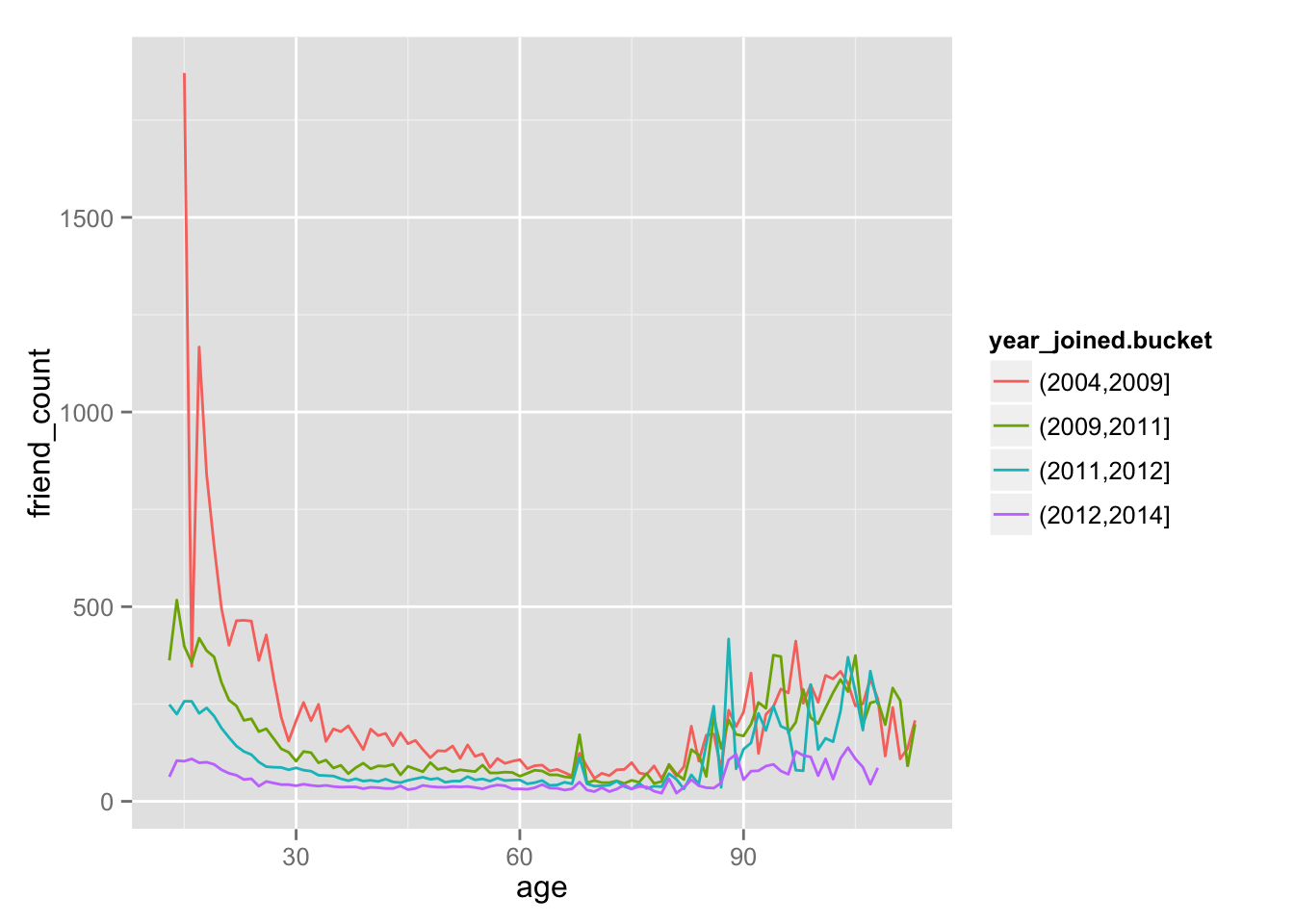In this plot, now we observe 3 variables, using x=friend_count, y=age, and year_joined.bucket as categorical variables. ***

### Plot the Grand Mean

Write code to do the following:

1. Add another geom_line to code below to plot the grand mean of the friend count vs age.

2. Exclude any users whose year_joined.bucket is NA.

3. Use a different line type for the grand mean.

As a reminder, the parameter linetype can take the values 0-6:

0 = blank, 1 = solid, 2 = dashed 3 = dotted, 4 = dotdash, 5 = longdash 6 = twodash

``````ggplot(aes(x = age, y = friend_count),
data = subset(pf, !is.na(year_joined.bucket)))+
geom_line(aes(color=year_joined.bucket), stat='summary', fun.y = mean)+
geom_line(fun.y = mean, stat='summary', linetype=2)``````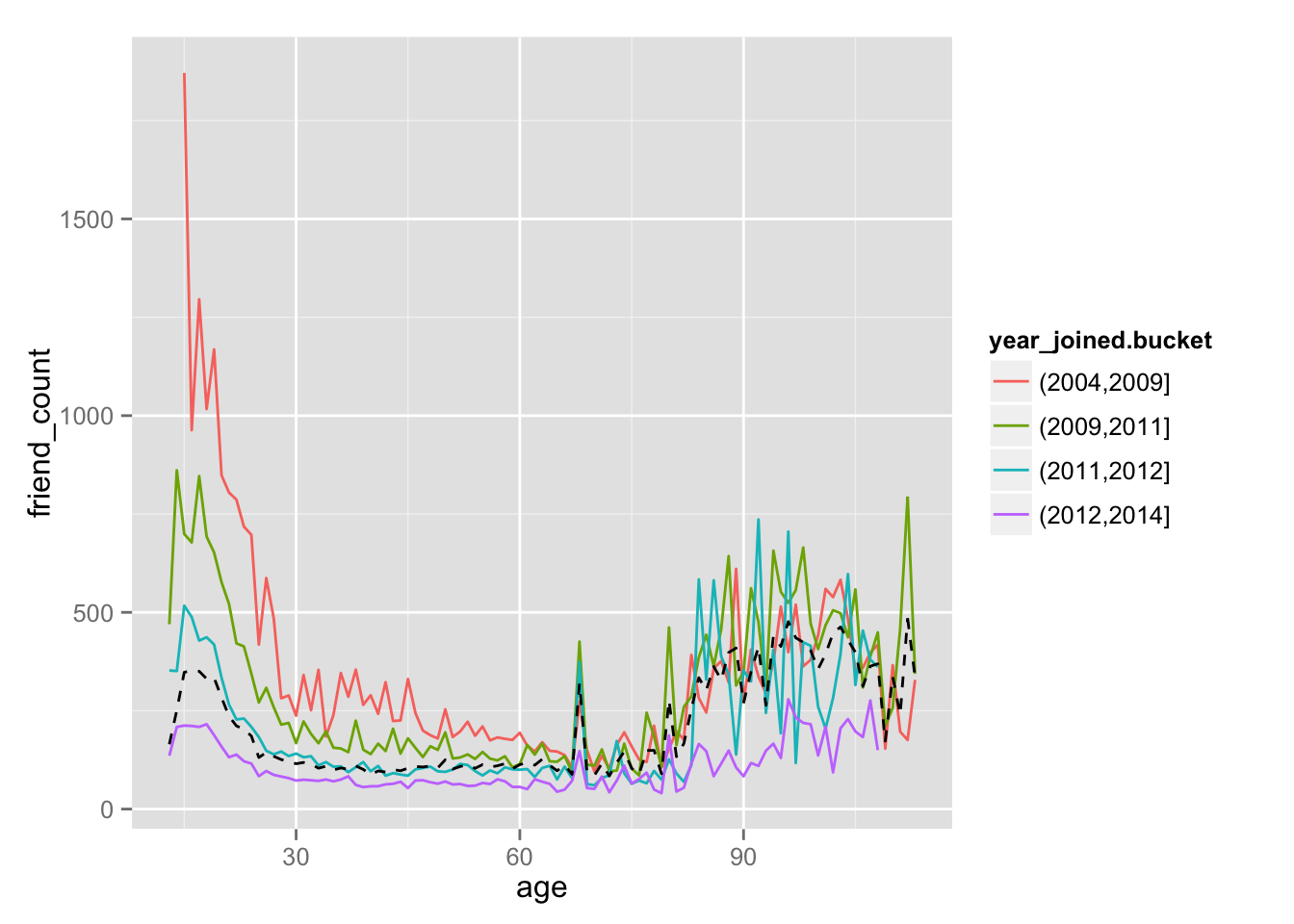### Friending Rate

• Now by plotting these, we know that the mean graph isn’t entirely artifact.
• So we want to ask another question. how many friend count the user have each day
``with(subset(pf, tenure > 1), summary(friend_count/tenure))``
``````##     Min.  1st Qu.   Median     Mean  3rd Qu.     Max.
##   0.0000   0.0775   0.2204   0.6069   0.5652 417.0000``````

### Friendships Initiated

in site longer, many friends more tenure intiate more friends

What is the median friend rate? .2205

What is the maximum friend rate? 417

Create a line graph of mean of friendships_initiated per day (of tenure) vs. tenure colored by year_joined.bucket.

You need to make use of the variables tenure, friendships_initiated, and year_joined.bucket.

You also need to subset the data to only consider user with at least one day of tenure.

``````ggplot(aes(x = tenure, y = friendships_initiated/tenure),
data = subset(pf, tenure>1))+
geom_line(aes(color=year_joined.bucket))``````